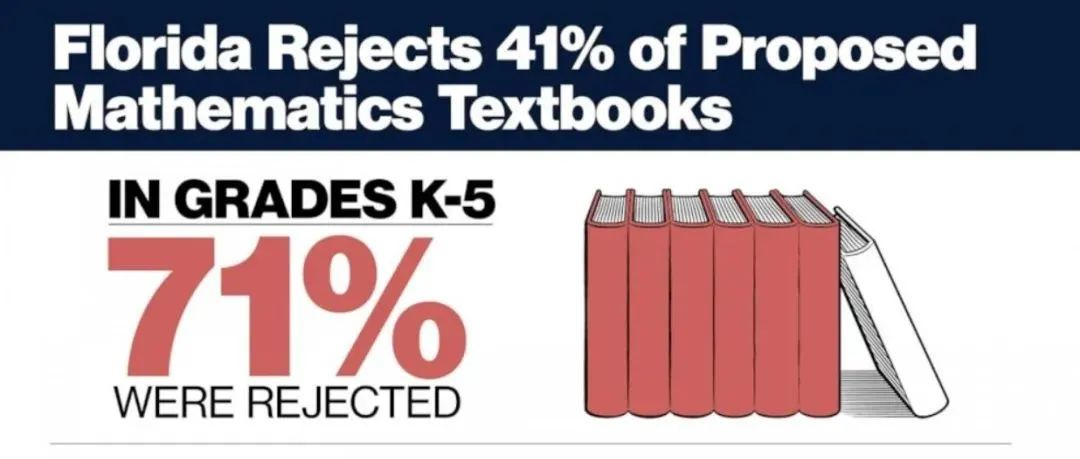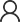# ARML美国区域数学联赛备赛辅导课程

ARML 主要包括区域赛(Local)、晋级挑战赛(power contest) 与国际赛(international)。区域赛在各个城市由各个学校组织，所有对数学感兴趣的学生都可以参加。晋级挑战赛邀请在区域赛中成绩优异的团队参加。国际赛主要在美国宾夕法尼亚大学、佐治亚州立大学、爱荷华州立大学和位于拉斯维加斯的内华达州立大学四所大学同时举办。Number Theory

Prime factorization; Number of divisors, Sum of divisors, Product of divisors; LCM and GCD

Euclidean Algorithm and Bezout's Theorem

Congruence and Divisibility

Chinese remainder theorem(CRT), Fermat’s little theorem, Euler’s function and theorem, Wilson's Theorem

Diophantine Equations

Algebra

Algebraic identities and Algebraic manipulations

Function Composition and Functional Equations (Induction and iteration Method)

Polynomials and Vieta's Theorem; Newton's Sum;

Fundamental inequalities, Cauchy inequality, other advanced inequalities

Rescursive Sequence; Characteristic Equation

Geometry

Basics in Geometry( Polygon; Area Method; The law of Cosine and the law of Sine)

Triangles, Centers of triangle; Menelaus's theorem, Ceva's theorem, Stewart Theorem

Probability and Statistic

Counting Principles (Sum Rules, Product Rules, Permutation and Combinations)

Geometric probability; Conditional Probability; Bayes Theorem

Logic reasoning (Pigeon Hole's Principle; Winning Strategy; Prove by contradiction; Principles of Inclusion and Exclusion)

Elementary Graph Theory; Coloring Problem and Labelling Method

College Topics

Limit, Differentiation, and simple Integration,

Simple Series and convergency test

Simple Group Theory### 北美驯鹿数学竞赛备赛辅导课程5. ### 剑桥大学2022年留学申请录取数据整体分析商务合作在线咨询